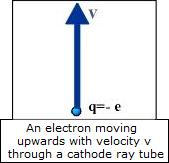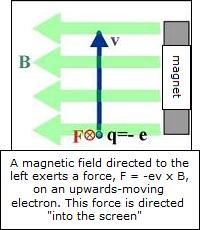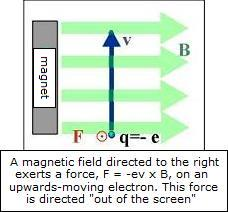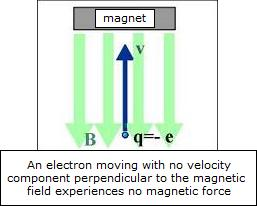### The force on a charged particle moving in a magnetic field is demonstrated with a cathode ray tube and a large bar magnet.

WATCH VIDEO

Teachable Topics:
• Magnetic fields
• Cathode ray tube

Theory:

A charged particle moving in a magnetic field experiences a force. If the magnetic field is B, and the particle's charge and velocity are q and v, respectively, then the magnetic force on the particle is F = qv x B. If the particle moving in an electric field is an electron, then q = -e, so the force on the electron is F = -ev xB.

The apparatus used in this demonstration is a cathode ray tube (CRT) facing upwards. The beam of electrons within the CRT travels upwards, so we'll take the direction of the velocity of the electron as being upward.

If a powerful magnet is brought near the beam of moving electrons from the right (north pole first), then the magnetic field will be oriented like in Figure 2.The cross product, v x B, must point out of the screen, but because the charge of the electron is negative, the force (F = -ev x B) must point into the screen. In the video below, the north pole of the magnet moving towards the beam from the right causes the beam to be deflected away from the camera.

Similarly, if the north pole of the magnet is brought in from the left, the direction of the magnetic field is reversed, and the force is as shown in the diagram below. In the video, this force pushes the electrons toward the camera.

In both of the previous situations, the magnetic field has been perpendicular to the direction of the electrons' velocity. What happens if the magnetic field and the velocity of the electrons lie along the same line? Bringing the magnet in from above means that the magnetic field is oriented as shown here:

The magnitude of the cross product v x B is |v x B| = |v||B|sinθ where θ = π is the angle between the antiparallel velocity and magnetic field vectors. The value of sinπ is zero (sinπ = 0), so the magnitude of the force on the electron is zero. The electron is not deviated from its path.Figure 2: Orientation of magnetic field upon encounter with magnet from the right.Figure 3: Orientation of magnetic field upon encounter with magnet from the left.Figure 4: Orientation of magnetic field upon encounter with magnet from above.

Apparatus
• a magnet
• a cathode ray tube

Procedure:

• Turn on the cathode ray tube (CRT) and allow it to warm up for a few minutes. You should see a green dot where the electrons strike the screen near its center. Bring the magnet's north pole towards the beam from one side. You will see the green dot move.
• Now center the magnet over top of the green dot and lower it towards the screen. As the magnet is lowered, you should see the dot stay in place, not being deflected in any direction.